### 如何找出正态分布的阈值 [问题点数：0分]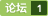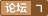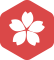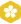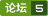Matlab信号提取、频谱分析、滤波、阈值设定、寻找极值点
from:    http://blog.sina.com.cn/s/blog_491b86bf0100noty.html好的作业题目可以激起人挑战的欲望，并指引着自己学习一系列的东西。可是这样的题目并不常见，上一次见到是什么时候我已经不记得了，昨天，一道让人忍不住叫好的作业题目摆在我的面前。先看题目，是某大学大四学生的课程作业：－－－－－－－题目Heart Beat Period Detecti...

本文参考博客使用opencv进行车牌提取及识别进行。程序部分为网上获取程序修改而来，并在其中加入了自己的注释和理解 采用python+opencv进行程序编写。 课程设计内容分享 程序下载请前往https://download.csdn.net/download/chenkz123/10841956 一个典型的车辆牌照识别系统一般包括以下4个部分：车辆图像获取、车牌定位、车牌字符分割...

% explain：计算主成分得分的马氏距离 % Dis_out——马氏距离输出 % erase_xuhao——异常样本的序号 % erase_N——异常点的总数 % data——输入数据，行为样本，列为特征 % weight——<em>阈值</em>调整权重系数，Threshold=mean(D)+weight*标准差 %lmd——前n个主成分对应的方差
torch中如何找出矩阵中元素之大于某个阈值的所有元素的下标？torch.find() ?

https://zhuanlan.zhihu.com/p/22142013这个文章，写的接地气，强烈推荐。http://www.cnblogs.com/rgvb178/p/6055213.html类似，但也值得一看。转自：http://blog.csdn.net/losteng/article/details/50833861激活函数是用来加入非线性因素的，因为线性模型的表达能力不够。常用激活函数...
[机器学习]有监督学习——通过特征学习来识别车牌号中的几个通用解决问题经验分享

R语言检测数据正态分布

<em>正态分布</em>（Normal distribution），也称“常态分布”，又名高斯分布（Gaussian distribution） 若随机变量X服从一个数学期望为μ、方差为σ^2的<em>正态分布</em>，记为N(μ，σ^2)。其概率密度函数为<em>正态分布</em>的期望值μ决定了其位置，其标准差σ决定了分布的幅度。当μ = 0,σ = 1时的<em>正态分布</em>是标准<em>正态分布</em>。 公式： 接下来画一个标准<em>正态分布</em>
【Python】提取列表内大于阈值提取位置
probs = np.array([[0, 0, 0, 0, 0, 1, 2], [1, 2, 2, 0, 0, 1, 2]]) a = np.array(probs &amp;gt;= 1, dtype='bool') c = np.nonzero(a) b = probs[a] print(probs, '\n',a, '\n', c, '\n', b,)结果[[0 0 0 0 0 1 2] [1 2...
OpenCV篇6---图像阈值（重点，很适用）

-
OpenCV下设置灰度直方图的阈值来对图像进行查找(查表)变换的源码

1. 基本形式{y=exy=e−x \left\{ \begin{array}{l} y=e^x\\ y=e^{-x} \end{array} \right. 2. 系数f(x)=⎧⎩⎨1θe−x/θ,0,x>0其他 f(x)=\left\{ \begin{array}{ll} \frac1\theta e^{-x/\theta},&x\gt 0\\ 0,&其他 \end{array} \right

opencv图像阈值设置
opencv python 图像<em>阈值</em>设置

<em>如何</em><em>找出</em>优质产品与关键词，<em>如何</em><em>找出</em>优质产品与关键词

Matlab中的正态分布概率函数
normcdf函数用来获得<em>正态分布</em>的概率分布函数； 也就是 normcdf(x)=Pr{Z≤x}Pr\{Z\leq x\}， 这里ZZ是均值为0，方差为1的标准正态随机变量. 若想获得均值为 μ\mu，方差为 σ\sigma的概率分布函数：normcdf(x,mu,sigma)即可.normpdf用来获得<em>正态分布</em>的概率密度函数. 用法与上同.
python中绘制roc曲线计算阈值
thresh.npy为正样例与反样例分别算出来的值，删去错误的预测样本，通过画roc曲线来计算<em>阈值</em> # -*- coding: utf-8 -*- import matplotlib.pyplot as plt import numpy as np from sklearn.metrics import roc_curve, auc import utils.config as config ...
R中验证正太分布检验及求取其方差和均值

<em>正态分布</em>（Normal distribution）又名高斯分布（Gaussian distribution），是一个在数学、物理及工程等领域都非常重要的概率分布，在统计学的许多方面有着重大的影响力。 若随机变量X服从一个数学期望为μ、标准方差为σ2的高斯分布，记为： X∼N(μ,σ2), 则其概率密度函数为 <em>正态分布</em>的期望值μ决定了其位置，其标准差σ决定了分布的幅度
opencv 提取轮廓大于某个阈值的图像
#include "stdafx.h" #include "cv.h" #include "highgui.h" #include "stdio.h" int main(int argc, char** argv) { const char* inputImage = "d:/3.jpg"; Mat img; int threshval =100; img = imr
matlab 实现双峰法全局阈值处理
img=imread('C:\Users\Administrator\Desktop\lwx.JPG'); im=rgb2gray(img); im_median=medfilt2(im); % 直方图 0-255 h = imhist(im_median); % 求极大值 粗略的算了一下 %IndMax=find(diff(sign(diff(count)))&amp;lt;0)+1; [cnt,x...

R语言代码：x &amp;lt;- seq(-2,2,0.1) par(mfrow=c(2,3)) plot(c(-2,2), c(0,8), type='n', main='exp(x)') lines(x, exp(x), col='red') plot(c(-2,2), c(0,8), type='n', main='exp(abs(x))') lines(x, exp(abs(x)), col...

matlab仿真正态分布曲线
% y= normpdf(x, mu,sigma)             mu --------期望， sigma-------------标准差 x=linspace(0,20); y1=normpdf(x,7,1); y2=normpdf(x,10,2); y3=normpdf(x,7.6,sqrt(0.8)); figure('color','w'); y=[y1;y2;y1.
SPSS如何验证是否符合正态分布
SPSS<em>如何</em>验证是否符合<em>正态分布</em> <em>正态分布</em>是T检验等统计分析的前提交通，本经验将介绍<em>如何</em>使用SPSS验证是否符合<em>正态分布</em>。 工具/原料 SPSS Statistics 测试数据集 方法/步骤 1 首先准备测试数据集，可以通过Excel或者Python等生成数据，本经验提供数据集如下： 81.09 81.73 82.38 83.02 83.67 ...

（分布检验问题）假设有n个随机数，检验这些随机数是否由高斯分布产生，方法如下： 1. 计算n个随机变量的平均值u； 2. 对n个随机变量排序，并计算相邻两个数的差dx； 3. 对第2个数到第n个数，计算z[i]=（x[i]-u)/dx; 4.求出max{z[i]},min{z[i]},将区间[min,max]划分n份，统计属于前i个区间的z[j]元素个数，即满足z[j]&amp;lt;=min+(...

1.图示法1.p-p图以样本的累积频率作为横坐标，以正太分布计算的响应累积概率作为纵坐标，把样本值表现为执教坐标系中的散点。若数据集服从正太分布，则样本点应围绕第一象限的对角线分布。1.2 QQ图以样本的分位数作为横坐标，以按照正太分布计算的相应分位点作为纵坐标，把样本表现为直角坐标系的散点。若服从正太分布，则样本点应该呈一条围绕第一象限对角线的直角。https://jingyan.baidu.c...

<em>正态分布</em>java.util.Random里的nextGaussian()，生成的数值符合均值为0方差为1的高斯/<em>正态分布</em>，即符合标准<em>正态分布</em>。 产生数字的范围：任何数都有可能，不过在0左右的数字较多。 产生N(a,b)的数：Math.sqrt(b)*random.nextGaussian()+a； 即均值为a，方差为b的随机数
python-检验33品种数据是否是正态分布
# -*- coding: utf-8 -*- """ Created on Thu Jun 22 17:03:16 2017 @author: yunjinqi E-mail:yunjinqi@qq.com Differentiate yourself in the world from anyone else. """ import pandas as pd import num

https://wenku.baidu.com/view/acc24dcf680203d8ce2f2469.html

by QZQ 基本 定义 性质 定性分析参数 标准<em>正态分布</em> 引理 分位点 相关的样本分布 2chi2分布 性质 上分位点 tt分布 上分位点 FF分布 上分位点 正态总体的样本均值与样本方差的分布 基本定义 若连续型随机变量X的概率密度为f(x)=12π‾‾‾√σe−(x−μ)22σ2,−∞<∞f(x)=\frac{1}{\sqrt{2\pi }\sigma } e ^{- \frac{(x-\

SPSS中如何检验数据是否服从正态分布（含举例）

1、Box–Muller算法 当x和y是两个独立且服从(0,1)均匀分布的随机变量时，则 Z1=cos(2πx)⋅–2ln(1–y)−−−−−−−−−√Z1=cos⁡(2πx)⋅–2ln⁡(1–y)Z_1=\cos (2\pi x) \cdot \sqrt { – 2\ln (1 – y)}Z2=sin(2πx)⋅–2ln(1–y)−−−−−−−−−√Z2=sin⁡(2πx)⋅–2l...

<em>正态分布</em>也叫常态分布，在我们后面说的很多东西都需要数据呈<em>正态分布</em>。下面的图就是<em>正态分布</em>曲线，中间隆起，对称向两边下降。     下面我们来看一组数据，并检验“期初平均分” 数据是否呈<em>正态分布</em>（此数据已在SPSS里输入好）     在SPSS里执行“分析—>描述统计—>频数统计表”(菜单见下图，英文版的可以找到相应位置)，然后弹出左边的对话框，变量
Python Dict找出value大于某值或key大于某值的所有项

【寒江雪】Box-Muler算法——均匀分布转正态分布
Box-Muler算法 ​ Box-Muller，一般是要得到服从<em>正态分布</em>的随机数，基本思想是先得到服从均匀分布的随机数再将服从均匀分布的随机数转变为服从<em>正态分布</em>。 ​ Box-Muller 算法隐含的原理非常深奥，但结果却是相当简单。它一般是要得到服从<em>正态分布</em>的随机数，基本思想是先得到服从均匀分布的随机数再将服从均匀分布的随机数转变为服从<em>正态分布</em>。 如果在 （0,1] 值域...

﻿﻿ 一、原理   OpenCV实现的贝叶斯分类器不是我们所熟悉的朴素贝叶斯分类器（Naïve Bayes Classifier），而是正态贝叶斯分类器（Normal Bayes Classifier），两者虽然英文名称很相似，但它们是不同的贝叶斯分类器。前者在使用上有一个限制条件，那就是变量的特征之间要相互独立，而后者没有这个苛刻的条件，因此它的适用范围更广。为了保持理论的系统性和完整性，
matlab 判断是否符合正态分布 lillietest
lillietest                    Lilliefors检验 常用语法                   [h,p]=lillietest(X) 说明                    返回值h为假设,只有0和1两种情况，h=0假设符合<em>正态分布</em>，h=1假设不符合<em>正态分布</em>                    返回值p为方差概率，也可以说事情的发生概率，p&amp;...

1 SPSS详细操作：正态转换的多种方法 2 SPSS教程：判断数据<em>正态分布</em>的超多方法！

1、对数变换 即将原始数据X的对数值作为新的分布数据：X’=lgX当原始数据中有小值及零时，亦可取X’=lg（X+1）还可根据需要选用X’=lg（X+k）或X’=lg（k-X）对数变换常用于（1）使服从对数<em>正态分布</em>的数据正态化。如环境中某些污染物的分布，人体中某些微量元素的分布等，可用对数<em>正态分布</em>改善其正态性。（2）使数据达到方差齐性，特别是各样本的标准差与均数成比例或变异系数CV接近于一个常数时...

C++产生正态分布
1.求均值 double sum = std::accumulate(std::begin(resultSet), std::end(resultSet), 0.0);   double mean =  sum / resultSet.size(); //均值  2.求方差 double accum  = 0.0;   std::for_each (std
matlab画正态分布图简单算法
matlab中的常用概率分布函数。 用matlab画<em>正态分布</em>图的代码： clear all； x=-4:0.1:4; y=normpdf(x,0,1); figure; plot(x,y);grid; legend('标准<em>正态分布</em>'); 结果如下 算出<em>正态分布</em>的alpha分位点。 用inv函数来实现：
R_数据正态分布检验

- 回顾 前面一直在讲述估计量的有效性（CRLB，线性模型），而没有提到假如估计量的方差没有达到CRLB，即是有效估计量不存在，但能够求出MVU估计量（假定存在）仍然是一个重要的事（可参考文章中的图片https://blog.csdn.net/GongPF/article/details/88715517）。因此，就提出了一般MVU估计。 - 主要使用的概念和方法 - 充分统计量（Su...
C语言--生成正态分布随机数

【学习笔记】统计学入门（4/7）——正态分布

Matlab实现正态分布
1）使用MatLab画出<em>正态分布</em>的概率密度函数图像。 x=[-10:0.01:10]; y=normpdf(x,0,1);%<em>正态分布</em>函数。 figure; axes1=axes('Pos',[0.1 0.1 0.85 0.85]); plot(x,y); set(axes1,'YLim',[-0.01 0.43],'XLim',[-3 3]); 图1： 2）验证概率密度函数在区间

<em>如何</em>用均匀分布随机数生成<em>正态分布</em>随机变量 前言 在Monte Carlo模拟技术中，许多地方都需要用到符合标准<em>正态分布</em>(高斯)的随机数来设计采样方案，因此了解<em>如何</em>用均匀分布随机数(实际上是均匀分布的伪随机数)来生成标准<em>正态分布</em>的随机数十分重要。本文将对这个最基本的问题做讨论，并提供c++11代码。 我们介绍两种算法: The Box–Muller transform 和 The Zig...
matlab 拟合正态分布

Matlab判断正态分布性检验

Stanford机器学习---第十一讲.异常检测

origin只画正态分布曲线，不画频率分布直方图的操作
1 选中ｙ　列＼

EA 建模工具下载
EA 是一种很好用的建模工具，支持与TFS进行交换 相关下载链接：[url=//download.csdn.net/download/lukelvquan/4603446?utm_source=bbsseo]//download.csdn.net/download/lukelvquan/4603446?utm_source=bbsseo[/url]
C#入门经典（第三版）part5下载
C#入门经典（第三版）C#入门经典（第三版）C#入门经典（第三版） 相关下载链接：[url=//download.csdn.net/download/ryuudenne/2069127?utm_source=bbsseo]//download.csdn.net/download/ryuudenne/2069127?utm_source=bbsseo[/url]